## CHAPTER XI The Bars Group

THIS group comprises those elements sometimes known as the Interperiodics. They occur in the pendulum diagram on the central line, alternately with the inert gases of the Star Group. They are all metals and have a maximum valence of eight.

When examined these elements were seen to have a striking configuration. Their general appearance is shown in Fig. 140. They consist of seven equal rods piercing a cube, three through the six middle points of its surfaces and four through its corners. There are therefore seven crossed bars whose directions in space are fixed by the cube. They may also be considered as consisting of fourteen half bars, all the half bars being identical. It should be clearly noted that there is no cube, nor outline of a cube to be seen in the element itself. The half-bars interlock in the centre of a sphere. The cube has been drawn simply to indicate the directions in space of the half-bars.

The elements in this group occur as closely associated sets of three. Three of these groups of three are known to science and a fourth group has been observed by clairvoyance and is here described. Within a group of three the difference between one member and its successor is 28 Anu, that is to say two extra Anu in each half-bar.

 ATOMIC No. ANU ELEMENT 14 BARS 26 1008 Iron 14 (2Fe14 + Fe16 + Fe28) 27 1036 Cobalt 14 (2Fe14 + Fe16 + 2Co11 + Co8) 28 1064 Nickel 14 (2Fe14 + Fe16 + 2Co11 + Ni.10) 44 1848 Ruthenium 14 (2Fe16 + 2Fe14 + 2Ru17 + 2Ru19) 45 1876 Rhodium 14 (2Fe16 + 2Fe14 + 2Rh20 + 2Rh17) 46 1904 Palladium 14 (2Rh17 + 2Pd15 + 2Pd17 + 2Pd19) - 2646 X 14 (3X30 + 3X28 + X15) - 2674 Y 14 (3X30 + 2Y29 + X28 + X15) - 2702 Z 14 (3X30 + 3Z31 + Cu10) 76 3430 Osmium 14 (4X30 + 3Z31 + Os32) 77 3458 Iridium 14 (4X30 + 2Ir26 + 2Ir27 + Ag21) 78 3486 Platinum 14 (4X30 + 2Ir26 + 2X28 + Ag21) 78 3514 Pt isotope 14 (4X30 + 2Ir27 + 2X28 + Ag21)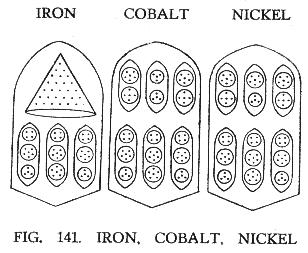FIG. 141. IRON, COBALT. NICKEL

• ATOMIC Nos. 26, 27, 28
• IRON, COBALT, NICKEL
• Owing to their similarity and mutual relationships it will be simplest to consider the groups of three elements together. Fig. 141.
• It will be noticed that each bar has two sections, and that the three lower sections in Iron, Cobalt and Nickel are identical (2Fe14 + Fe16). In the upper sections Iron has a cone of twenty-eight Anu, Fe28, while Cobalt and Nickel have each three ovoids, and of these the middle ones alone differ, and that only in their upper globes, this globe having four Anu in Cobalt and six in Nickel.
• As explained previously, the groups of Anu are in three dimensional space. The ovoids within each bar revolve round the central axis of the bar, remaining parallel with it, while each spins on its own axis; the Iron cone spins round as though impaled on the axis.
• Iron = 14(2Fe14 + Fe16 + Fe28)
• 14 bars of 72 Anu = 1008 Anu
• Total = 1008 Anu
• Number weight 1008 / 18 = 56.00tk
• Cobalt = 14(2Fe14 + Fe16 + 2Co11 + Co8)
• 14 bars of 74 Anu = 1036 Anu
• Total = 1036 Anu
• Number weight 1036 / 18 = 57.555tk
• Nickel = 14(2Fe14 + Fe16 + 2Co11 + Ni.10)
• 14 bars of 76 Anu = 1064 Anu
• Total = 1064 Anu
• Number weight 1064 / 18 = 59.11tk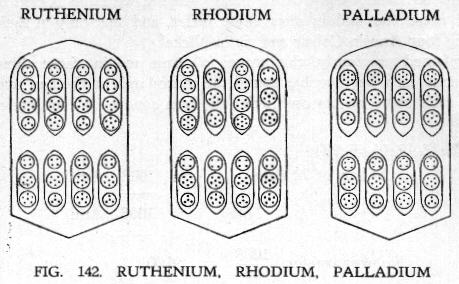• ATOMIC Nos. 44, 45, 46
• The next sub-group, Ruthenium, Rhodium and Palladium, is formed on the same pattern. Fig. 142. It will be observed that each bar contains eight ovoids, instead of the six of Cobalt and Nickel. Ruthenium and Palladium have the same number of Anu in their upper ovoids, although in Ruthenium a triplet and quartet replace the septet of Palladium. In Ruthenium and Rhodium the lower ovoids are identical, though Ruthenium has the order: sixteen, fourteen, sixteen, fourteen; and Rhodium: fourteen, sixteen, fourteen, sixteen. One constantly asks oneself: What is the significance of these minute changes?
• Ruthenium = 14 (2Fe16 + 2Fe14 + 2Ru17 + 2Ru19)
• 14 bars of 132 Anu = 1848 Anu
• Total = 1848 Anu
• Number weight 1848 / 18 = 102.666tk
• Rhodium = 14 (2Fe16 + 2Fe14 + 2Rh20 + 2Rh17)
• 14 bars of 134 Anu = 1876 Anu
• Total = 1876 Anu
• Number weight 1876 / 18 = 104.22tk
• Palladium = 14 (2Rh17 + 2Pd15 + 2Pd17 + 2Pd19)
• 14 bars of 136 Anu = 1904 Anu
• Total = 1904 Anu
• Number weight 1904 / 18 = 105.777tk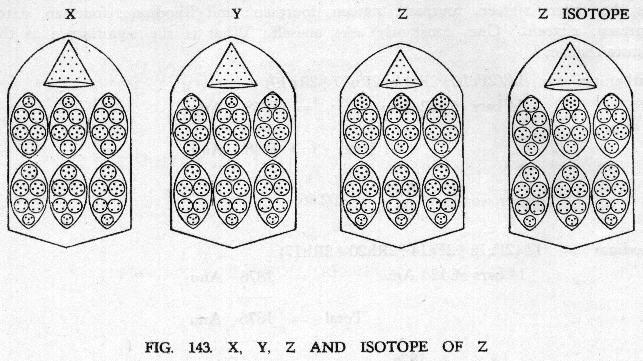FIG. 143. X, Y, Z AND ISOTOPE OF Z

• ATOMIC No. -
• ELEMENTS X, Y, Z
• This Group fills in the gap in the periodic table. In each of the fourteen bars there are two sections, each containing three ovoids, and a cone - Fig. 143.
• The lower sections in each of these elements are similar, each consisting of three ovoids of thirty Anu, X30.
• X contains three groups X28 in its upper section and then a cone of fifteen Anu.
• Y is similar, save that it contains only one X28 but adds two groups of twenty-nine Anu, Y29.
• Z contains three groups of thirty Anu in its upper section. An isotope of Z was observed, differing only by one Anu in each bar. The cone in Z has ten Anu.
• X = 14(3X30 + 3X28 + X15)
• 14 bars of 189 Anu = 2646 Anu
• Total = 2646 Anu
• Number weight 2646 / 18 = 147.00tk
• Y = 14(3X30 + 2Y29 + X28 + X15)
• 14 bars of 191 Anu = 2674 Anu
• Total = 2674 Anu
• Number weight 2674 / 18 = 148.555tk
• Z = 14(3X30 + 3Z31 + Cu10)
• 14 bars of 193 Anu = 2702 Anu
• Total = 2702 Anu
• Number weight 2702 / 18 = 150.11tk
• Z isotope = 14(3X30 + 2Y29 + Z31 + X15)
• 14 bars of 194 Anu = 2716 Anu
• Total = 2716 Anu
• Number weight 2716 / 18 = 150.888tk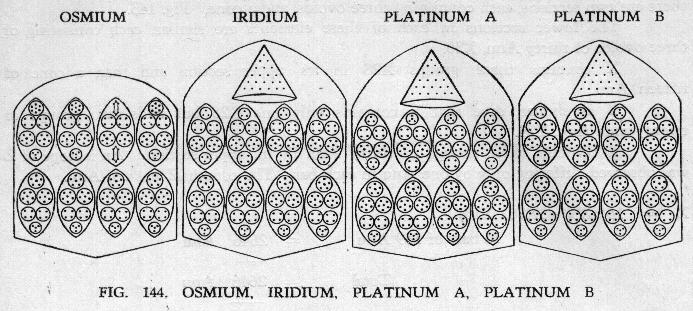FIG. 144. OSMIUM, IRIDIUM, PLATINUM A, PLATINUM B

• ATOMIC Nos. 76, 77, 78
• OSMIUM, IRIDIUM, PLATINUM
• The fourth group, Osmium, Iridium and Platinum is, of course, more complicated in its composition, but its builders succeed in preserving the bar form, gaining the necessary increase by additional contained spheres within the ovoids. Osmium has one peculiarity: the ovoid Os32 takes the place of the axis, in the upper half of the bar, and the three ovoids, Z31, revolve round it. In the lower half, the four ovoids. X30, revolve round the central axis. Fig. 144.
• Osmium.
• Each section contains four ovoids very similar to those already met with in X, Y and Z the lower four being identical with X30.
• Osmium = 14 (4X30 + 3Z31 + Os32)
• 14 bars of 245 Anu = 3430 Anu
• Total = 3430 Anu
• Number weight 3430 / 18 = 190.555tk
• Iridium.
• It will be noticed that the lower sections of the bars are identical in all the members of this sub-group, each of the four ovoids containing thirty Anu, X30. The upper ring of ovoids in Iridium and Platinum A are also identical but for the substitution, in Platinum A, of a quartet for a triplet in the second and third ovoids; their cones are identical, containing twenty-one Anu, like those of Silver and Tin.
• Iridium = 14 (4X30 + 2Ir26 + 2Ir27 + Ag21)
• 14 bars of 247 Anu = 3458 Anu
• Total = 3458 Anu
• Number weight 3458 / 18 = 192.11tk
• Platinum.
• In Platinum, we have observed two forms. Platinum A and Platinum B, the latter having two spheres of four Anu in the place of the two triplets. It may well be that what we have called Platinum B is not a variety of Platinum, but a new element, the addition of two Anu in a bar being exactly that which separates the other elements within each of the sub-groups.
• Platinum A = 14 (4X30 + 2Ir26 + 2X28 + Ag21)
• 14 bars of 249 Anu = 3486 Anu
• Total = 3486 Anu
• Number weight 3486 / 18 = 193.666tk
• Platinum B = 14 (4X30 + 2Ir27 + 2X28 + Ag21)
• 14 bars of 251 Anu = 3514 Anu
• Total = 3514 Anu
• Number weight 3514 / 18 = 195.22tk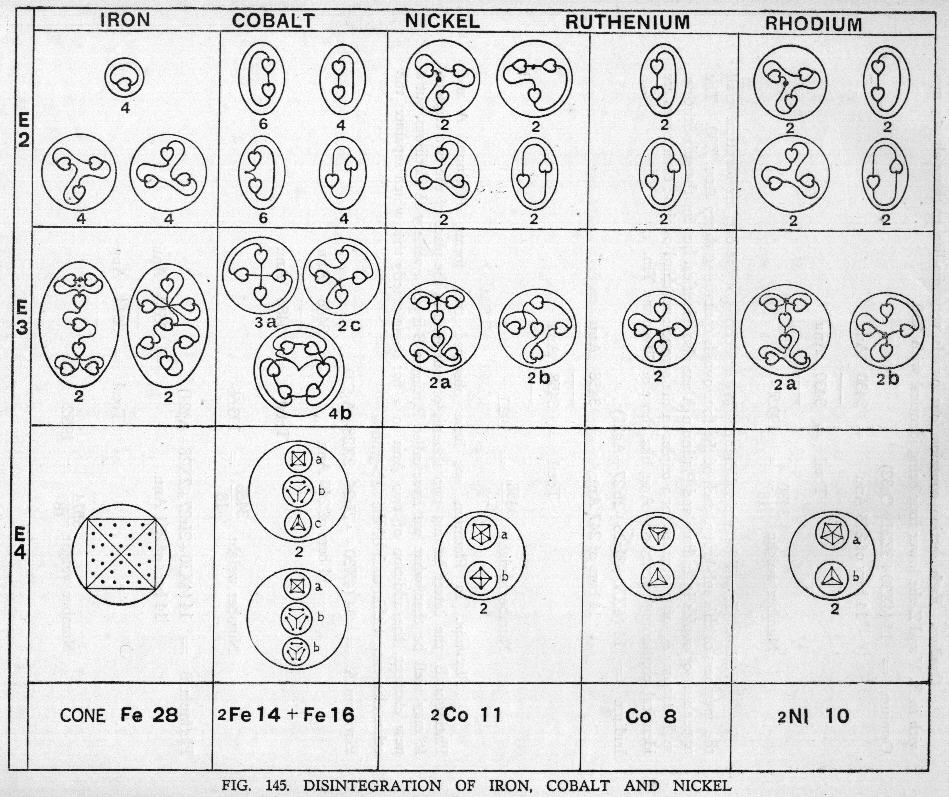FIG. 145. THE BARS GROUP

### DISINTEGRATION OF THE BARS GROUP

• DISINTEGRATION OF IRON. COBALT, NICKEL
• Iron.
• The 14 bars of iron break asunder on the E4 level and each sets free its contents, a cone and three ovoids. Fig. 145.
• The cone of twenty-eight Anu becomes a four-sided figure with seven Anu in each face. On the E3 level this cone gives four septets and these are reduced to triplets and units on the E2 level.
• The ovoids Fe 14 and Fe16 show crystalline contents on the E4 level and become spherical in shape. On the E3 level these three spheres give four sestets and two quartets of one type and three of another. They reduce to duads of various types on the E2 level.
• Cobalt.
• The three lower ovoids in Cobalt are identical with those in Iron. The cone is replaced by three upper ovoids, two being Co.11 and one Co8. These all become spheres on the E4 level. On the E3 level the three lower ovoids behave as in Iron, while the Co.11 gives a sextet and a quintet and the Co8 two quartets. On the E3 level triplets and duads are formed as shown in Fig. 145.
• Nickel.
• The three lower ovoids are identical with those of Iron and Cobalt and disintegrate in the same way.
• Of the three upper ovoids, two are Co.11. The third, Ni.10, contain a sextet and a quartet and forms a sphere on the E4 level. On the E3 level these give a sextet and a quartet and on the E2 level triplets and duads. All these can be followed in Fig. 145.

Fig. 146 shows the Bars Group in a condensed form, from which the relationships can be studied.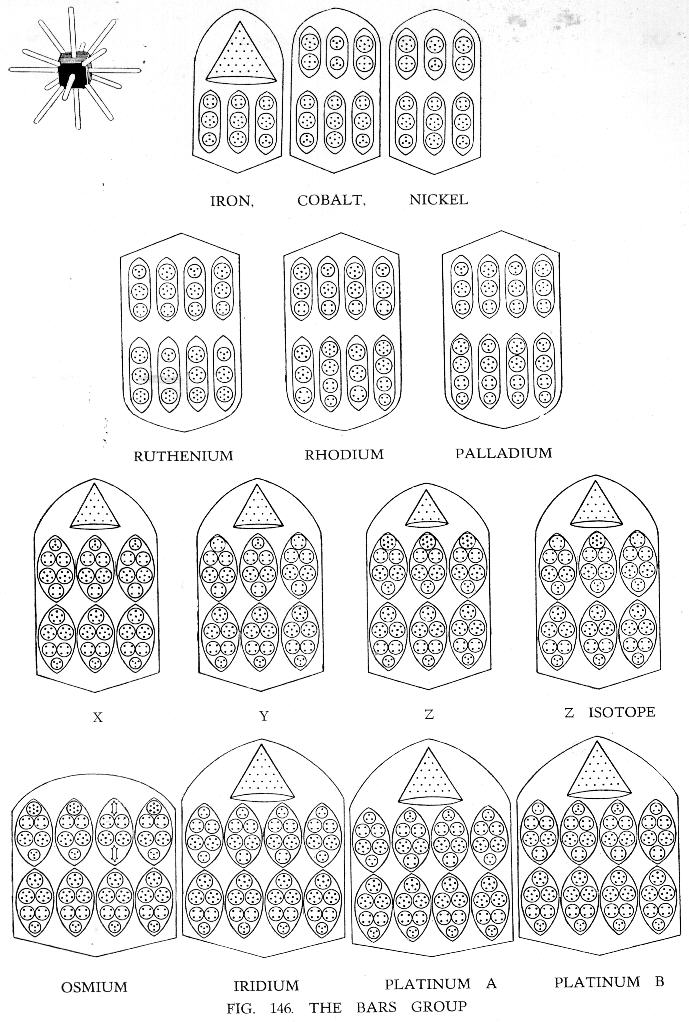FIG. 146. THE BARS GROUP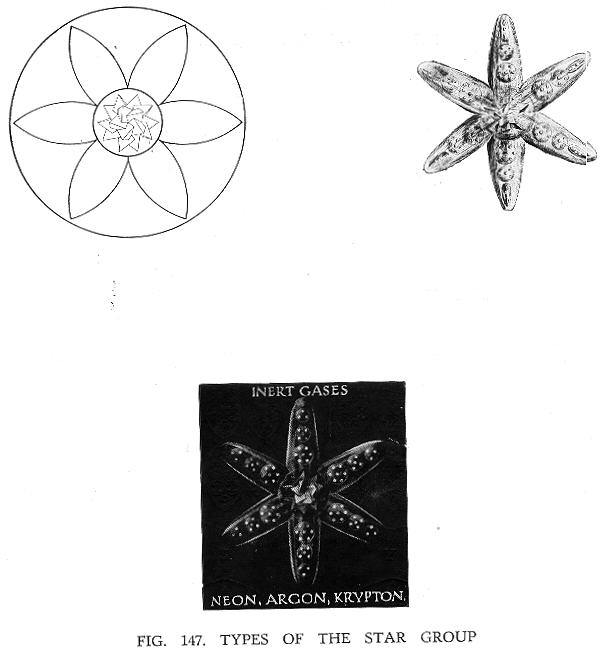FIG. 147. TYPES OF THE STAR GROUP

Next: Chapter XII   The Star Group

## Acknowledgements

 TPH Twilight Archive

HTML validation by:

 W3C online validation service for HTML 4.0

spell-checked, files merged, some errors corrected,
reset in HTML 4.0 in March, April, May 2000
last revision November 2003